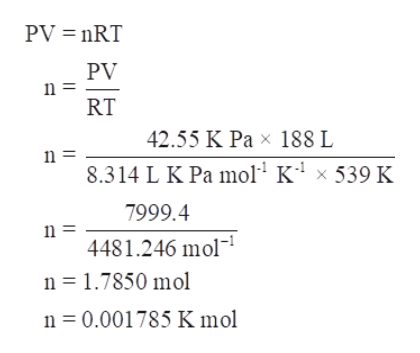# AND EQUILIBRIUMKINECalculating the reaction rate of one reactant from that of anotherDENitric acid is a key industrial chemical, largely used to make fertilizers and explosives. The first step in its synthesis is the oxidation of ammonia. In this reaction,gaseous ammonia reacts with dioxygen gas to produce nitrogen monoxide gas and water.Suppose a chemical engineer studying a new catalyst for the oxidation of ammonia reaction finds that 188, liters per second of dioxygen are consumed when thereaction is run at 266. °C and 0.42 atm. Calculate the rate at which nitrogen monoxide is being produced. Give your answer in kilograms per second. Round youranswer to 2 significant digits.kgx10x 5?CheckExplanationPrivacy2019 McGraw-Hill Education. All Rights Reserved. Terms of Use

Question
7 views

Calculate the rate at which nitrogen monoxide is being reduced give your answer in kilograms per second round your answer to two significant digits.

check_circle

Step 1

Given,

Temperature of the reaction = 266°C = 266 + 273 K = 539 K

Pressure of the reaction = 0.42 atm = 42.55 K Pa

Volume of dioxygen = 188 litres

Gas Constant, R = 8.314 L K Pa K-1 mol-1

According to ideal gas equation,help_outlineImage TranscriptionclosePV nRT PV n = RT 42.55 K Pa x 188 L 8.314 L K Pa mol K4 x 539 K 7999.4 4481.246 mol n 1.7850 mol n 0.001785 K mol fullscreen
Step 2

Now the value of n=0.001785 K mol which is equal to the 17.8 g moles of nitrogen produced.

The reaction of the mixture is –

Therefore, the product of the...

### Want to see the full answer?

See Solution

#### Want to see this answer and more?

Solutions are written by subject experts who are available 24/7. Questions are typically answered within 1 hour.*

See Solution
*Response times may vary by subject and question.
Tagged in

### Chemistry